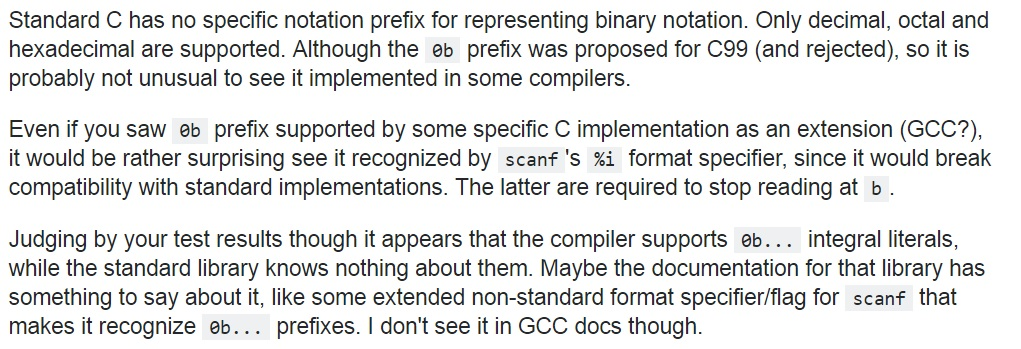# How to assign binary value in a variable directly?

We have assigned Hexadecimal, Decimal, and Octal values in a variables in our many previous solutions.

Decimal value can be assigned directly (int a=100 ;), Octal value can be assigned by using "0" notation (int b=0144 ;) and Hexadecimal value can be assigned by using "0X" or "0x" notation (int c=0x64 ;).

First consider the following example, which is assigning Decimal, Octal and Hexadecimal values in variable and printing their values in Decimal format.

```#include <stdio.h>
int main()
{
int a=100;
int b=0144;
int c=0x64;

printf("a= %d\n",a);
printf("b= %d\n",b);
printf("c= %d\n",c);

return 0;
}
```

Output

```    a= 100
b= 100
c= 100
```

The values of a, b and c are 100 because, 0144 is the octal value of 100 and 0x64 is the Hexadecimal value of 100.

## How to assign Binary value in a variable?

Binary value can be assigned in a variable by using "0b" notation (we can say it format specifier too), this is a new feature which was introduced in C99 (not a standard feature, some compilers may not support this feature).

Let’s consider the following program

```#include <stdio.h>
int main()
{
int num=0b1010;
printf("Num: %d\n",num);
return 0;
}
```

Output

```    Num: 10
```

Remember: Read this post before using 0b notation to represent binary value
http://stackoverflow.com/questions/11597863/why-does-scanfi-a-not-take-binary-like-0b101